Question Pool Dual Nature of Radiation and Matter

# Dual Nature of Radiation and Matter - SAMAGRA Question Pool & Answers | Class 12

Kerala Syllabus SAMAGRA SCERT SAMAGRA Question Pool for Class 12 Physics Dual Nature of Radiation and MatterQn 1.

Momentum of a photon with wavelength λ is………….

p = h/

Get Free Study Materials + 1 Week Free Trial of BrainsPrep Class 12 Tuition

Qn 2.

The graph shows the variation of KE with frequency of incident radiations for two surfaces A and B.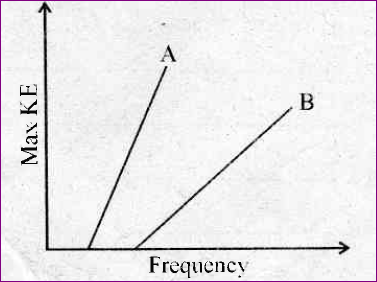1. Which of the metal has greater work function? For which of the metals will stopping potential be more for the same frequency of incident radiation?

2. The work function of cesium metal is 2.14 eV. When light of frequency 6 x 1014 Hz incident on the metal surface , what is the maximum KE of the photoelectrons and stopping potential?

Get Free Study Materials + 1 Week Free Trial of BrainsPrep Class 12 Tuition

Qn 3.

Given below is the graph between frequency (υ) of the incident light and maximum kinetic energy (Ek) of emitted photoelectrons.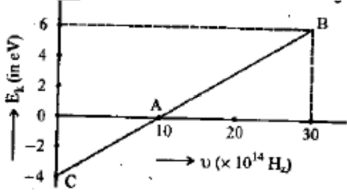Find the values of i. threshold frequency and ii. Work function from the graph.

1. Threshold frequency = 10 x 1014 Hz

2. Work function

Get Free Study Materials + 1 Week Free Trial of BrainsPrep Class 12 Tuition

Qn 4.

When photons incident on a metallic surface electric current is produced. The work function of the metal is given by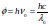.The graph given below shows the variation in stopping potential with frequency ν0 of the incident radiation of the materials A and B.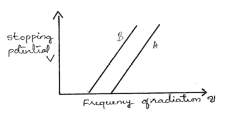1. Which of the material has higher work function?

2. Which material is more photosensitive?

3. What happens to the slope of the graph when intensity of radiation increases? Why?

Get Free Study Materials + 1 Week Free Trial of BrainsPrep Class 12 Tuition

Qn 5.

a) What is de Broglie hypothesis? Write the formula for de Broglie wavelength.

b) Calculate the de Broglie wavelength associated by an electron accelerated through a potential difference of 100 volts.

Get Free Study Materials + 1 Week Free Trial of BrainsPrep Class 12 Tuition

Qn 6.

All photoelectrons are not emitted with the same energy as the incident photons. Why?

A part of the photon energy is used as the work function to eject an electron. So the energy of the electrons emitted will be less than

Get Free Study Materials + 1 Week Free Trial of BrainsPrep Class 12 Tuition

Qn 7.

Write Einstein’s photoelectric equation and explain the terms involved in it

Get Free Study Materials + 1 Week Free Trial of BrainsPrep Class 12 Tuition

Qn 8.

Work function of a metal is the

(a) energy required by an electron to get absorbed in the metal surface.

(b) minimum energy required by an electron to escape from the metal surface

(c) energy required by an electron to be retained by a metal surface.

(d) maximum energy required by an electron to escape from the metal surface.

(b) minimum energy required by an electron to escape from the metal

Get Free Study Materials + 1 Week Free Trial of BrainsPrep Class 12 Tuition

Qn 9.

When light falls on a certain metals photo electrons are generated.

1. Express the phenomenon in terms of equation.

2. Explain the terms used.

Get Free Study Materials + 1 Week Free Trial of BrainsPrep Class 12 Tuition

Qn 10.

Electron can undergo diffraction just like waves. What is the wavelength of an electron accelerating in a potential difference of 54 V?

Get Free Study Materials + 1 Week Free Trial of BrainsPrep Class 12 Tuition

Qn 11.

(a) The work function a metal is 6eV.If two photons each having energy 4 eV strike with the metal surface.

(i) will the emission be possible? (ii) Why?

(b) The waves associated with matter is called matter waves. Let λe and λp be the de Broglie wavelengths associated with electron and proton respectively. If they are

accelerated by same potential, then

1. λe> λp ii) λp> λe iii) λp= λe iv)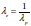Get Free Study Materials + 1 Week Free Trial of BrainsPrep Class 12 Tuition

Qn 12.

Photoelectric current depends on the intensity of incident light.

1. The maximum current emitted by a photoelectric material is called......

(i) Emitter current (ii) Collector current (iii) Saturation current (iv) Peak current

1. Work function of cesium and platinum are 2.14 eV and 5.65 eV respectively. Which one of the metals has higher threshold wavelength? Justify.

Get Free Study Materials + 1 Week Free Trial of BrainsPrep Class 12 Tuition

Qn 13.
1. The wavelength of matter waves is called de Broglie Wavelength.

1. An α -particle, a proton and an electron having de Broglie wavelengths λα , λp and λe respectively are moving with the same momentum. Then

i. λα> λp> λe ii) λp> λe> λα iii) λα= λp = λe  iv) λp= λe ≠ λα

b) The de Broglie wavelength of a ball of mass 0.12 Kg is 2.76 x 10-34 m. Calculate the speed of the ball. [ h= 6.625 x 10-34 Js]

Get Free Study Materials + 1 Week Free Trial of BrainsPrep Class 12 Tuition

Qn 14.

The graph shows photoelectric current with anode potential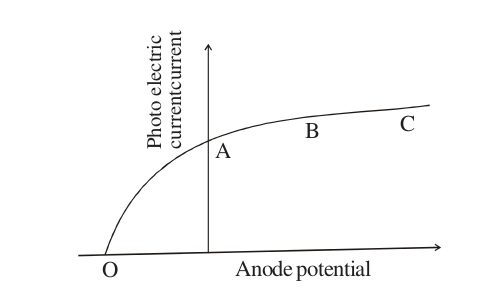a. The potential at ‘O’ is called

(i) accelerating potential (ii) retarding potential (iii) stopping potential (iv) saturation potential

b. Why current becomes constant in the region BC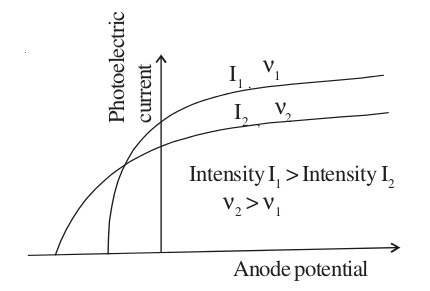a. Stopping potential

b. At a particular anode potential all the electrons from the cathode

Get Free Study Materials + 1 Week Free Trial of BrainsPrep Class 12 Tuition

Qn 15.

Which of the following element shows lowest work function ?

( a ) Cs                 ( b ) K              ( c ) Na                ( d ) Ca

. a )

Get Free Study Materials + 1 Week Free Trial of BrainsPrep Class 12 Tuition

Qn 16.

Is work function is same for all metals ?

No , work function is difference for different

Get Free Study Materials + 1 Week Free Trial of BrainsPrep Class 12 Tuition

Qn 17.

What is work function ?

The minimum energy required for an electron to eject from the metal

Get Free Study Materials + 1 Week Free Trial of BrainsPrep Class 12 Tuition

Qn 18.

What are the different types of electron emission ? , Explain

i) Thermionic emission: By suitably heating, sufficient thermal energy

can be imparted to

Get Free Study Materials + 1 Week Free Trial of BrainsPrep Class 12 Tuition

Qn 19.

What do you mean by photo electric emission ?

The emission of electrons from the metal surface by incidenting

Get Free Study Materials + 1 Week Free Trial of BrainsPrep Class 12 Tuition

Qn 20.

The scientist who succeeded in finding the the value of specific charge of an electron ?

R A

Get Free Study Materials + 1 Week Free Trial of BrainsPrep Class 12 Tuition

Qn 21.

What is the the value of e/m of electron ?

The value of e/m is 1.76 ×

Get Free Study Materials + 1 Week Free Trial of BrainsPrep Class 12 Tuition

Qn 22.

Which of the following is the representation of specific charge of an electron

( a ) e                    ( b ) m                 ( c ) e/m                          ( d ) m/e

( c )

Get Free Study Materials + 1 Week Free Trial of BrainsPrep Class 12 Tuition

Qn 23.

What do you mean by stopping potential ?

The retarding potential applied to a metal surface in order to stop the emitted photo

Get Free Study Materials + 1 Week Free Trial of BrainsPrep Class 12 Tuition

Qn 24.

Which of the following factors depends on kinetic energy of the emitted photoelectron

( a ) Mass of the metal                                              ( b ) Intensity of the incident photon

( c ) Nature of the incident photon                             ( d ) Frequency of the incident photon

( d ) Frequency of the incident

Get Free Study Materials + 1 Week Free Trial of BrainsPrep Class 12 Tuition

Qn 25.

Explains Einstein’s photoelectric equation ?

The maximum kinetic energy of the emitted photoelectron is

Get Free Study Materials + 1 Week Free Trial of BrainsPrep Class 12 Tuition

Qn 26.

Mention some examples of metals showing photoelectric effect .

Alkali metals such as lithium, sodium, potassium, caesium and rubidium were sensitive

even

Get Free Study Materials + 1 Week Free Trial of BrainsPrep Class 12 Tuition

Qn 27.

Define photoelectric effect ?

The phenomenon of emssion of electrons from the surface a metal by the incidence of certain types of radiations called photoelectric